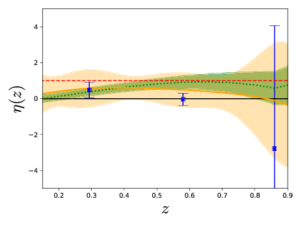Authors:
##### Noemi Frusciante (U. Lisbon (main)), Simone Peirone (Leiden U.), Santiago Casas (CEA Paris-Saclay), Nelson A. Lima (U. Heidelberg, ITP)
Journal: Submitted to PRD
Year: 10/2018

## Abstract

In the context of the effective field theory of dark energy (EFT) we perform agnostic explorations of Horndeski gravity. We choose two parametrizations for the free EFT functions, namely a power law and a dark energy density-like behaviour on a non trivial Chevallier-Polarski-Linder background. We restrict our analysis to those EFT functions which do not modify the speed of propagation of gravitational waves. Among those, we prove that one specific function cannot be constrained by data, since its contribution to the observables is below the cosmic variance, although we show it has a relevant role in defining the viable parameter space. We place constraints on the parameters of these models combining measurements from present day cosmological datasets and we prove that the next generation galaxy surveys can improve such constraints by one order of magnitude. We then verify the validity of the quasi-static limit within the sound horizon of the dark field, by looking at the phenomenological functions μ and Σ, associated respectively to clustering and lensing potentials. Furthermore, we notice up to 5% deviations in μ,Σ with respect to General Relativity at scales smaller than the Compton one. For the chosen parametrizations and in the quasi-static limit, future constraints on μ and Σ can reach the 1% level and will allow us to discriminate between certain models at more than 3σ, provided the present best-fit values remain.

## Model-independent reconstruction of the linear anisotropic stress

 Authors: Ana Marta Pinho,  Santiago Casas, Luca Amendola Journal: Accepted for JCAP Year: 05/2018 Download: Inspire| Arxiv

## Abstract

In this work, we use recent data on the Hubble expansion rate H(z), the quantity fσ8(z) from redshift space distortions and the statistic Eg from clustering and lensing observables to constrain in a model-independent way the linear anisotropic stress parameter η. This estimate is free of assumptions about initial conditions, bias, the abundance of dark matter and the background expansion. We denote this observable estimator as ηobs. If ηobs turns out to be different from unity, it would imply either a modification of gravity or a non-perfect fluid form of dark energy clustering at sub-horizon scales. Using three different methods to reconstruct the underlying model from data, we report the value of ηobs at three redshift values, z=0.29,0.58,0.86. Using the method of polynomial regression, we find ηobs=0.57±1.05, ηobs=0.48±0.96, and ηobs=0.11±3.21, respectively. Assuming a constant ηobs in this range, we find ηobs=0.49±0.69. We consider this method as our fiducial result, for reasons clarified in the text. The other two methods give for a constant anisotropic stress ηobs=0.15±0.27 (binning) and ηobs=0.53±0.19 (Gaussian Process). We find that all three estimates are compatible with each other within their 1σ error bars. While the polynomial regression method is compatible with standard gravity, the other two methods are in tension with it.